# Drawing plots with Pandas

Standard

In this new entry, we will see the plotting capabilities of Pandas and how to mix it with Matplotlib.

In the introduction to plotting libraries, we mentioned that Pandas has some plotting capabilities. By default, these capabilities are somehow limited, but come in preety handly. Let’s see an example, using data on fuel economy for some popular car models downloaded from here. We will draw a line plot showing the average fuel economy (in miles per gallon, therefore the higher the better) per number of cylinders for all the models. For that, we first group by the number of cylinders and get the mean. The mean() method returns a DataFrame object over which we can use the loc[] method. Note that this is not very effective for very large datasets, because we are calculating the averages of all columns to use only two of them; but the code for taking two is out of the scope of this entry. You can find more about that here.

In :
```%matplotlib inline
import pandas as pd
import matplotlib.pyplot as plt
fedata = pd.read_csv('mpg.csv', index_col=0)
fedata.groupby('cyl').mean().loc[:,['hwy','cty']].plot()
plt.show()
```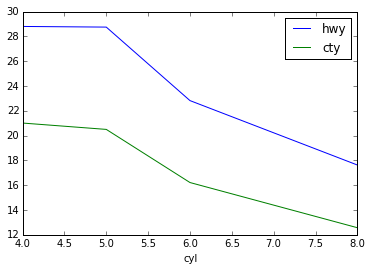We can conclude that the higher number of cylinders, the lower the fuel economy. But I’d rather have a car with 6 cylinders rather than one with 5.5, wouldn’t you? This graphic is misleading in the sense that there are no cars with 5.5 cylinders. We must modify this graphic in order to add some accuracy. Now, Pandas plotting capabilities are based on Matplotlib. According to the DataFrame.plot() documentation, we can also pass it keyword arguments that will be passed to the underlying Matplotlib plot() function. What does this mean for us? Basically that we can treat a DataFrame.plot() or a Series.plot() as a normal Matplotlib plot(). So everything we did in the previous blog entry can be used here.

In :
```fedata.groupby('cyl').mean().loc[:,['hwy','cty']].plot(linewidth=2, colors=['k','r'])

plt.legend(['Highway','City'])
plt.xlabel('Fuel economy (MPG) per number of Cylinders')
plt.axis([3,9,0,30])

ax = plt.axes()
ax.spines['bottom'].set_linewidth(2)
ax.spines['left'].set_linewidth(2)
ax.spines['top'].set_visible(False)
ax.spines['right'].set_visible(False)

ax.xaxis.set_ticks_position('bottom')
ax.yaxis.set_ticks_position('left')
ax.xaxis.set_ticks(fedata.loc[:,'cyl'].unique())
ax.xaxis.set_ticklabels(fedata.loc[:,'cyl'].unique())
ax.tick_params(labelsize=14)

ax.yaxis.grid(True, which='major')
ax.xaxis.grid(True, which='major')

plt.show()
```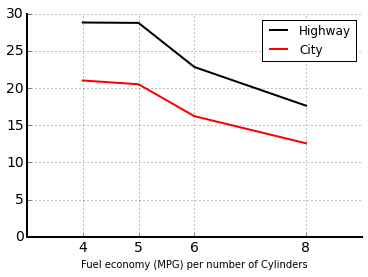The only new element in the code above is in lines 15 and 16, where we have used the unique() method to extract all the distinct values of the number of cylinders.

We’ll keep on drawing graphs to see the capabilities of Pandas. But we’ll keep it simple and ugly, otherwise the decoration lines would saturate our code.

Let’s say we want to see the relation between the engine displacement and the fuel economy. For that we can draw a scatter plot:

In :
```fedata.plot(kind='scatter', y='displ', x='cty')
fedata.plot(kind='scatter', y='displ', x='hwy')
plt.show()
```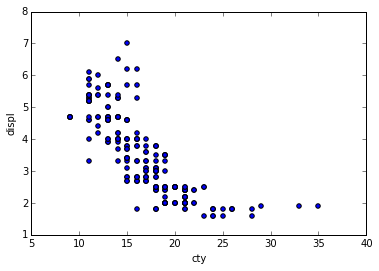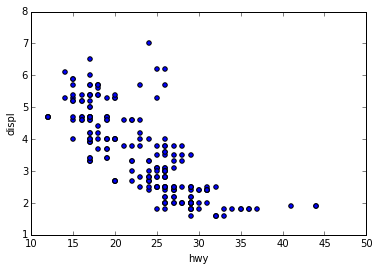Generally speaking, we can say that the higher the displacement, the lower the mileage. We can also do an analysis classifying the cars by class and drawing a barplot:

In :
```fedata.groupby('class').mean().loc[:,['cty','hwy']].plot(kind='bar')
plt.show()
```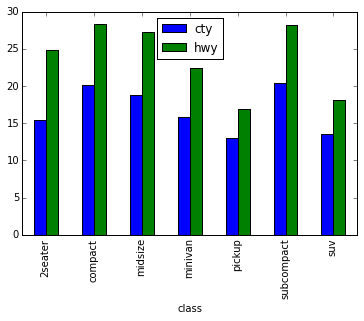Compared with the default barplot provided by Matplotlib, this one already has some customzations; for instance, the columns are centered over the labels and the labels are rotated for reading them more easily. This definitely shows that the plotting subsystem in Pandas is designed for quick and easy visualizations that help in the process of data analysis.

As always, there is a lot more to talk and show about the plotting capabilities of Pandas. Nevertheless, with what is shown here, we can conclude that this part of the library is mostly designed to help us for data visualization more than to create distributable graphics. We still can do that using the decoration processes of Matplotlib; with its advantages (customization, availability of functions, documentation …) and disadvantages (difficulty, code bloating, …).

This site uses Akismet to reduce spam. Learn how your comment data is processed.